# Electric Power Distribution 1 Electric Power Distribution Electric

• Slides: 24Electric Power Distribution 1 Electric Power Distribution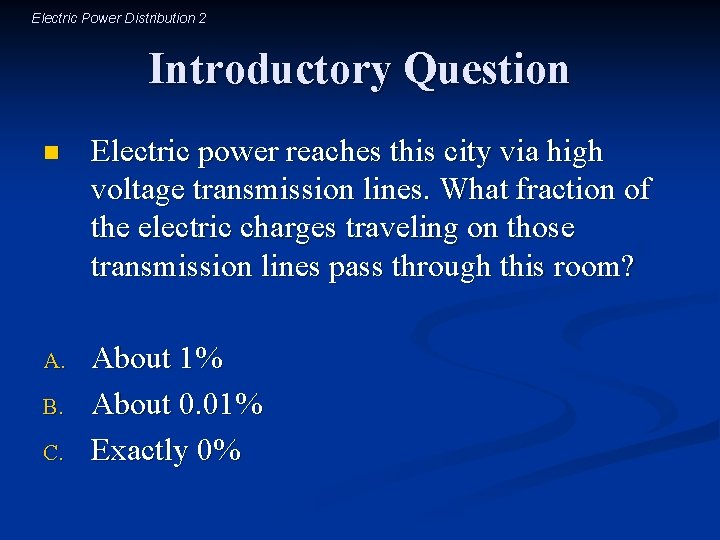Electric Power Distribution 2 Introductory Question n Electric power reaches this city via high voltage transmission lines. What fraction of the electric charges traveling on those transmission lines pass through this room? A. About 1% About 0. 01% Exactly 0% B. C.Electric Power Distribution 3 Observations about Electric Power Distribution Household electricity is alternating current (AC) n Household voltages are typically 120 V or 240 V n Power is distributed at much higher voltages n Power transformers are common n Power substations are less common n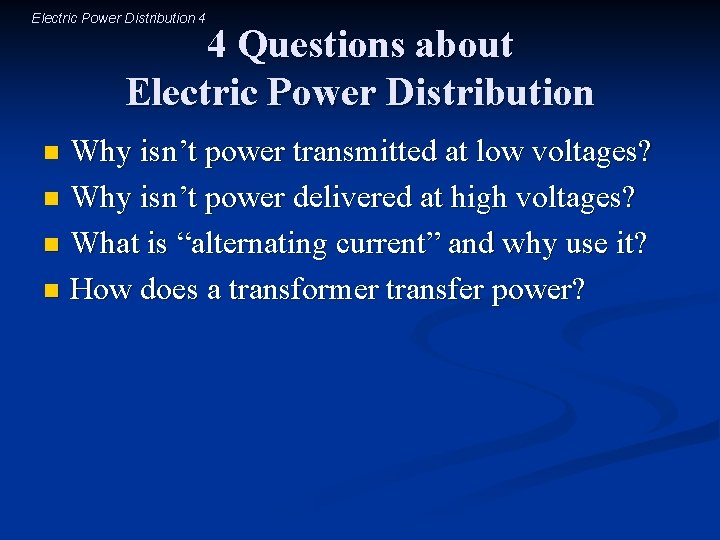Electric Power Distribution 4 4 Questions about Electric Power Distribution Why isn’t power transmitted at low voltages? n Why isn’t power delivered at high voltages? n What is “alternating current” and why use it? n How does a transformer transfer power? n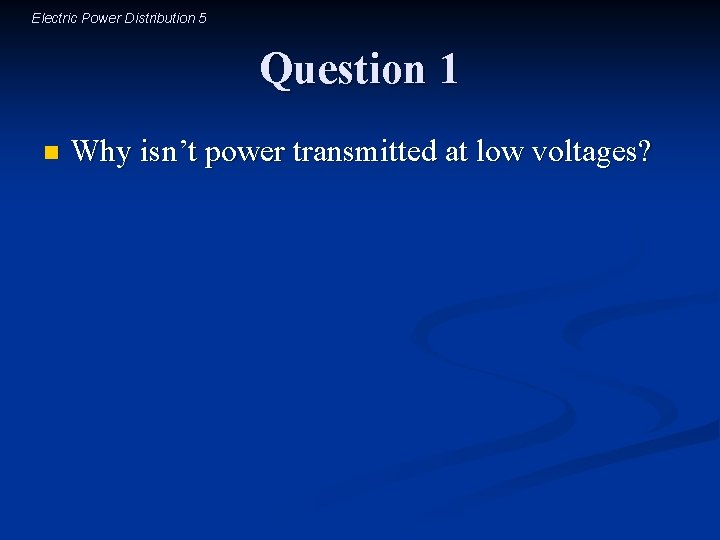Electric Power Distribution 5 Question 1 n Why isn’t power transmitted at low voltages?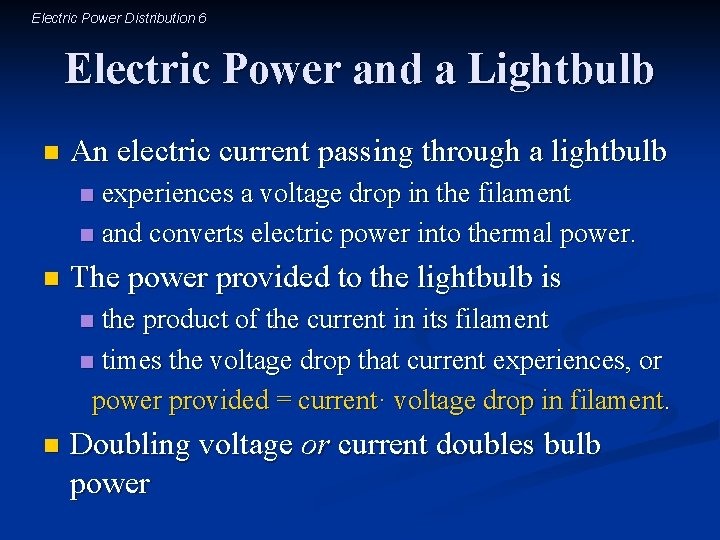Electric Power Distribution 6 Electric Power and a Lightbulb n An electric current passing through a lightbulb experiences a voltage drop in the filament n and converts electric power into thermal power. n n The power provided to the lightbulb is the product of the current in its filament n times the voltage drop that current experiences, or power provided = current· voltage drop in filament. n n Doubling voltage or current doubles bulb power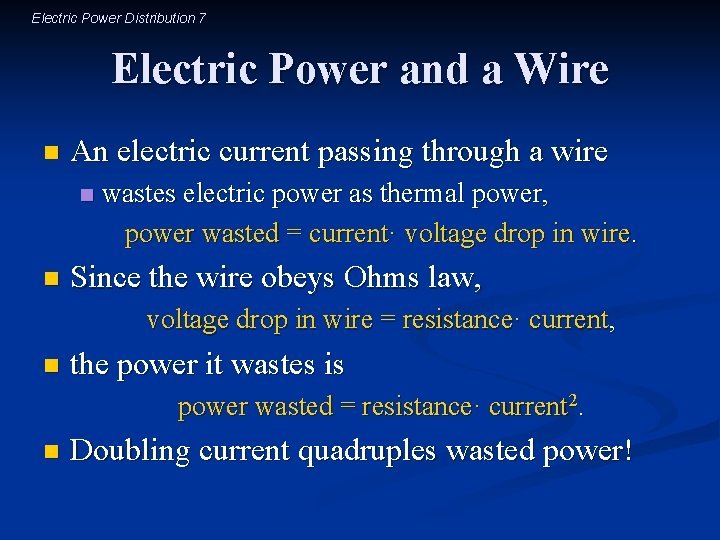Electric Power Distribution 7 Electric Power and a Wire n An electric current passing through a wire n n wastes electric power as thermal power, power wasted = current· voltage drop in wire. Since the wire obeys Ohms law, voltage drop in wire = resistance· current, n the power it wastes is power wasted = resistance· current 2. n Doubling current quadruples wasted power!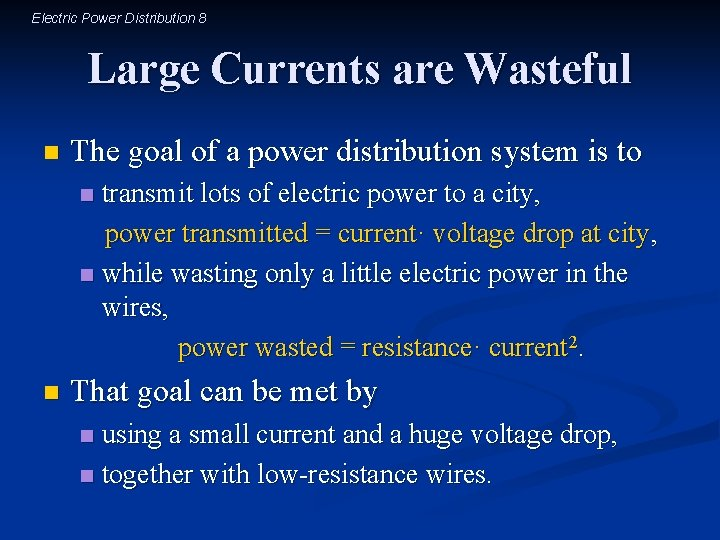Electric Power Distribution 8 Large Currents are Wasteful n The goal of a power distribution system is to transmit lots of electric power to a city, power transmitted = current· voltage drop at city, n while wasting only a little electric power in the wires, power wasted = resistance· current 2. n n That goal can be met by using a small current and a huge voltage drop, n together with low-resistance wires. n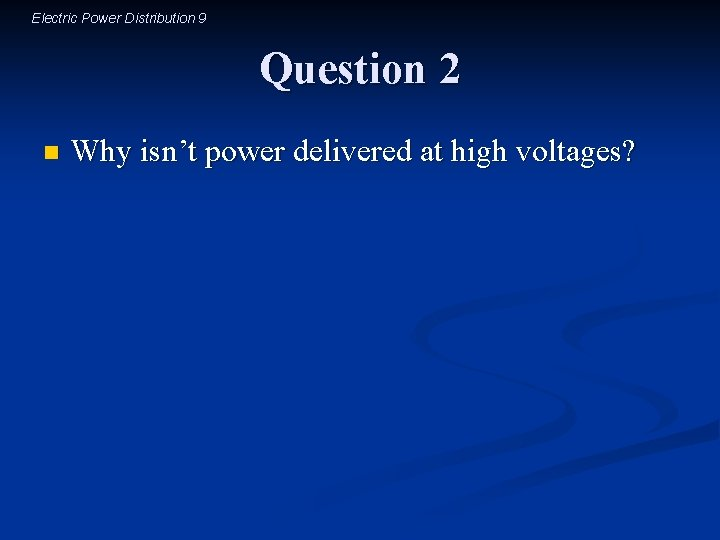Electric Power Distribution 9 Question 2 n Why isn’t power delivered at high voltages?Electric Power Distribution 10 High Voltages are Dangerous n When large voltage drops are available, charges tend to jump toward the lower voltage n and currents tend to flow through unexpected paths. n n High-voltage electrical power in a home is a spark hazard, n a fire hazard, n and a shock hazard. n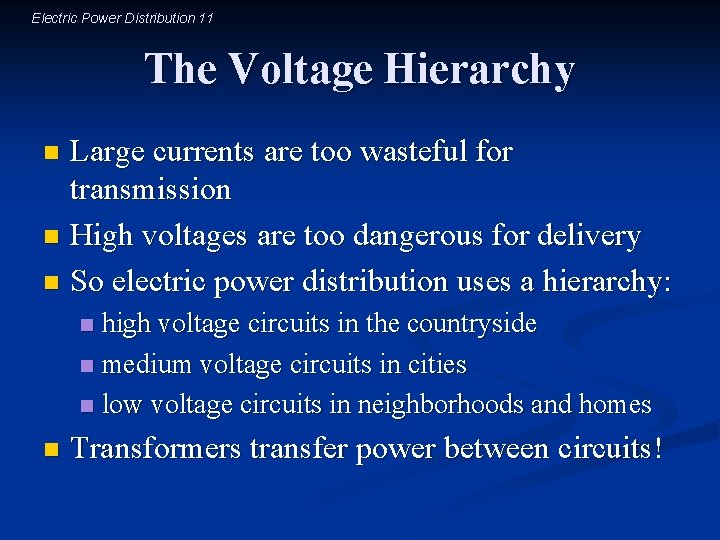Electric Power Distribution 11 The Voltage Hierarchy Large currents are too wasteful for transmission n High voltages are too dangerous for delivery n So electric power distribution uses a hierarchy: n high voltage circuits in the countryside n medium voltage circuits in cities n low voltage circuits in neighborhoods and homes n n Transformers transfer power between circuits!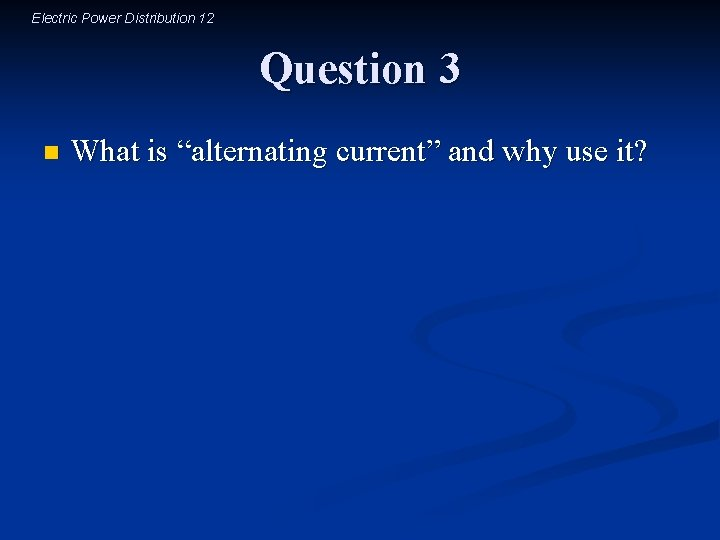Electric Power Distribution 12 Question 3 n What is “alternating current” and why use it?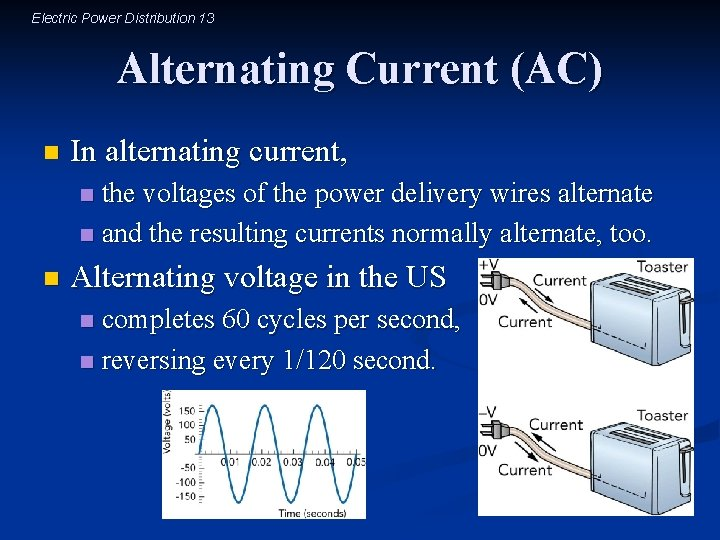Electric Power Distribution 13 Alternating Current (AC) n In alternating current, the voltages of the power delivery wires alternate n and the resulting currents normally alternate, too. n n Alternating voltage in the US completes 60 cycles per second, n reversing every 1/120 second. nElectric Power Distribution 14 AC and Transformers AC has little effect on simple electric devices (e. g. , lightbulbs, space heaters, toasters), n but it’s a nuisance for electronic devices (e. g. , computers, televisions, sound systems). n AC produces alternating magnetic fields n and transformers use alternating magnetic fields n to move power between AC circuits! nElectric Power Distribution 15 Question 4 n How does a transformer transfer power?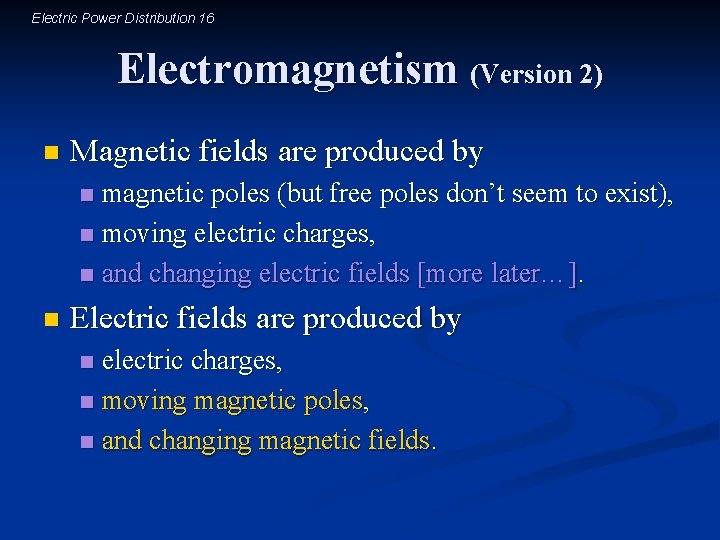Electric Power Distribution 16 Electromagnetism (Version 2) n Magnetic fields are produced by magnetic poles (but free poles don’t seem to exist), n moving electric charges, n and changing electric fields [more later…]. n n Electric fields are produced by electric charges, n moving magnetic poles, n and changing magnetic fields. n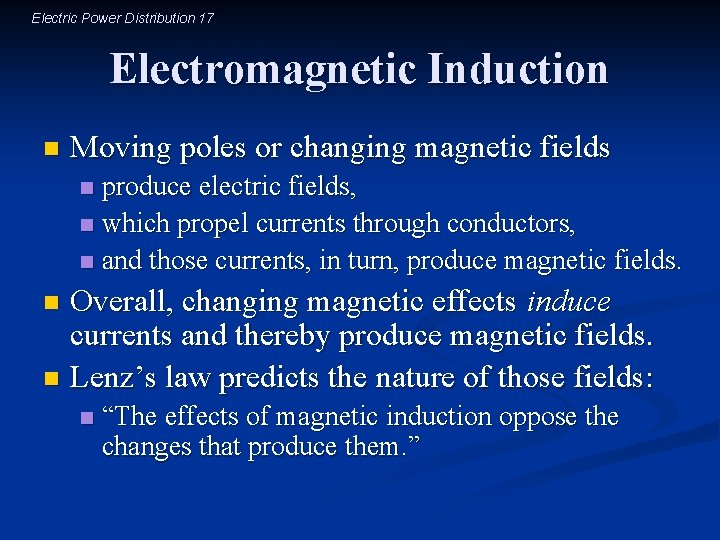Electric Power Distribution 17 Electromagnetic Induction n Moving poles or changing magnetic fields produce electric fields, n which propel currents through conductors, n and those currents, in turn, produce magnetic fields. n Overall, changing magnetic effects induce currents and thereby produce magnetic fields. n Lenz’s law predicts the nature of those fields: n n “The effects of magnetic induction oppose the changes that produce them. ”Electric Power Distribution 18 Transformer Alternating current in one circuit can induce an alternating current in a second circuit n The transformer n transfers power between the circuits n but it doesn’t transfer any charges between the circuits nElectric Power Distribution 19 Current and Voltage Power arriving in the primary circuit must equal power leaving in the secondary circuit n Since power is the product of voltage· current, n a transformer can obey energy conservation n while exchanging voltage for current n or current for voltage! n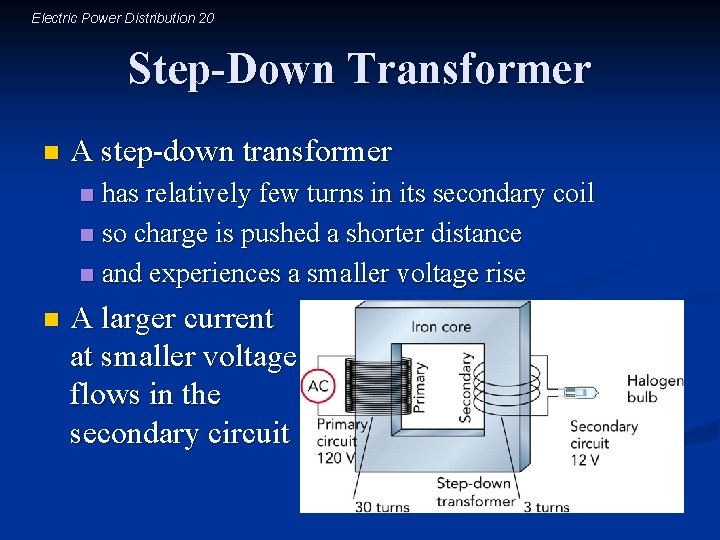Electric Power Distribution 20 Step-Down Transformer n A step-down transformer has relatively few turns in its secondary coil n so charge is pushed a shorter distance n and experiences a smaller voltage rise n n A larger current at smaller voltage flows in the secondary circuitElectric Power Distribution 21 Step-Up Transformer n A step-up transformer has relatively many turns in its secondary coil n so charge is pushed a longer distance n and experiences a larger voltage rise n n A smaller current at larger voltage flows in the secondary circuitElectric Power Distribution 22 Power Distribution System A step-up transformer increases voltage for efficient long-distance transmission n A step-down transformer decreases voltage for safe delivery to communities and homes n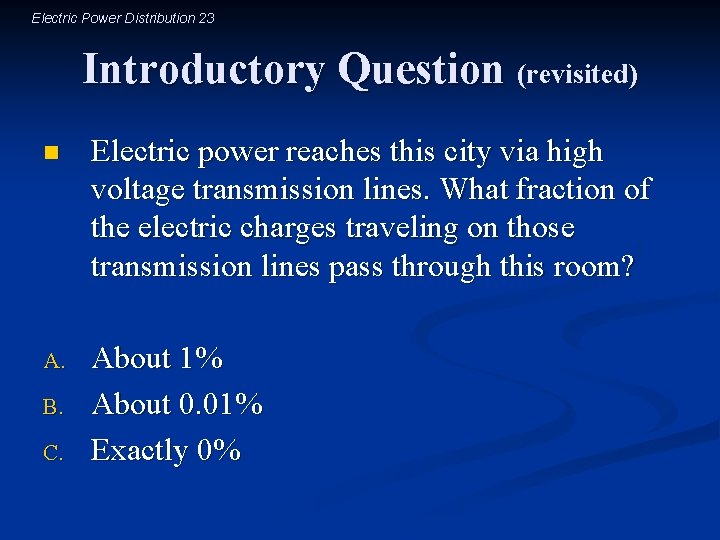Electric Power Distribution 23 Introductory Question (revisited) n Electric power reaches this city via high voltage transmission lines. What fraction of the electric charges traveling on those transmission lines pass through this room? A. About 1% About 0. 01% Exactly 0% B. C.Electric Power Distribution 24 Summary about Electric Power Distribution Electric power is transmitted at high voltages n Electric power is delivered at low voltages n Transformers transfer power between circuits n Transformers require AC power to operate n The power distribution system is AC n